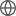Old Web
English
We present the discovery of copious molecular gas in the halo of cid_346, a $z=2.2$ quasar studied as part of the SINFONI survey for Unveiling the Physics and Effect of Radiative feedback (SUPER). New Atacama Compact Array (ACA) CO(3-2) observations detect a much higher flux (by a factor of $14\pm5$) than measured on kiloparsec scales ($r\lesssim8$ kpc) using previous snapshot Atacama Large Millimeter/submillimeter Array data. Such additional CO(3-2) emission traces a structure that extends out to $r\sim200$ kpc in projected size, as inferred through direct imaging and confirmed by an analysis of the uv visibilities. This is the most extended molecular circumgalactic medium (CGM) reservoir that has ever been mapped. It shows complex kinematics, with an overall broad line profile (FWHM $= 1000$ km/s) that is skewed towards redshifted velocities up to at least $v\sim1000$ km/s. Using the optically thin assumption, we estimate a strict lower limit for the total molecular CGM mass observed by ACA of $M_{mol}^{CGM}>10^{10}~M_{\odot}$. There is however room for up to $M^{CGM}_{mol}\sim 1.7\times 10^{12}$ $M_{\odot}$, once optically thick CO emission with $\alpha_{\rm CO}=3.6$ $\rm M_{\odot}~(K~km~s^{-1}~pc^2)^{-1}$ and $L^{\prime}_{CO(3-2)}/L^{\prime}_{CO(1-0)}=0.5$ are assumed. Since cid_346 hosts quasar-driven ionised outflows and since there is no evidence of merging companions or an overdensity, we suggest that outflows may have played a crucial rule in seeding metal-enriched, dense gas on halo scales. However, the origin of such an extended molecular CGM remains unclear.